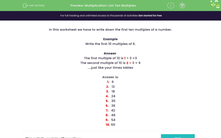# Find the first 10 multiples of a number

In this worksheet, students write down the first ten multiples of the given numbers.Key stage:  KS 2

Curriculum topic:   Number: Multiplication and Division

Curriculum subtopic:   Use Multiplication/Division Facts (3, 4 and 8)

Popular topics:   Times Table worksheets

Difficulty level:#### Worksheet Overview

In this activity, we will be learning about finding the first ten multiples of a number.

A multiple is the product we get when we multiply a number.

So, some multiples of 5 would be 5, 10, 15, 20...

It's important to remember that when we multiply a number by 1, it stays the same.

So, multiples are not always larger than the number itself.

We can find the first ten multiples of a number by skip counting or using times tables facts.

Let's try an example together.

Example

Write the first 10 multiples of 6.

The first multiple of 10 is 1 × 3 =3

The second multiple of 10 is 2 × 3 = 6

The first ten multiples of 6 are as follows:

1.   6

2.   12

3.   18

4.   24

5.   30

6.   36

7.   42

8.   48

9.   54

10. 60

### What is EdPlace?

We're your National Curriculum aligned online education content provider helping each child succeed in English, maths and science from year 1 to GCSE. With an EdPlace account you’ll be able to track and measure progress, helping each child achieve their best. We build confidence and attainment by personalising each child’s learning at a level that suits them.

Get started